# Numbers of Months (elapsed time) since a month when consecutive zeros are startedHi Anaplaners,

I would like to figure out numbers of months elapsed since consecutive zeros have started. (please see the attached).

For instance, in the attached file, as sales of product BBB is zero since July 2019(instead of April 2019), I would like to get "4"(months) given current period is Oct 2019.

Regards,

Greg

Tagged:

•There is, as ever, a much simpler approach

1. Set up a time lookup module that holds the value of the current period.  Format the line item as time period - month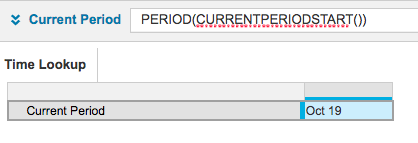2. Create three additional line items in your data module.  We will be utilising a little known technique that allows us to "reset" a cumulate count based on a boolean parameter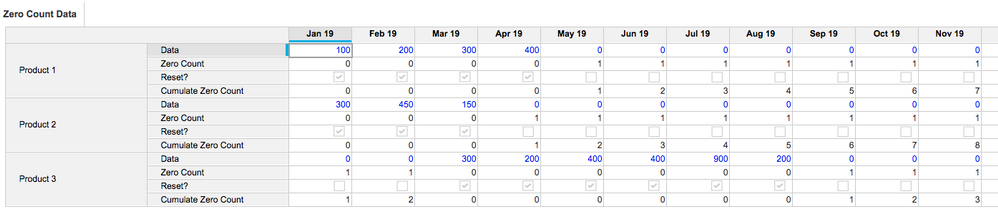Zero Count = IF Data > 0 THEN 0 ELSE 1

Reset? = Zero Count = 0

Cumulate Zero Count = CUMULATE(Zero Count, Reset?)

3. Then you can link this to the summary module using the line item from 1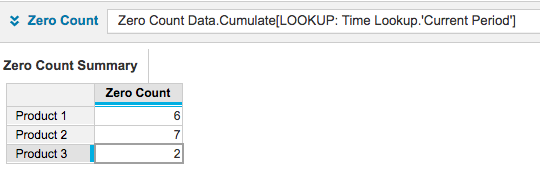This allows for data within the run of zeros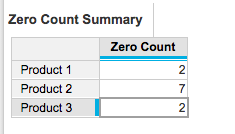As a general note, remember that all calculations that only refer to time should be calculated in a time settings module rather than in multi-dimensional modules.

E.g. START() <= CURRENTPERIODSTART()

and

PERIOD(CURRENTPERIODSTART())

I hope this hepls

David

•What you have proposed is perfectly working. But another provided simpler solution, which I have to accept as a solution for this problem.

•Hi Greg,

#1. Create a line item (Zero Month), set format as month and set Summary as last non blank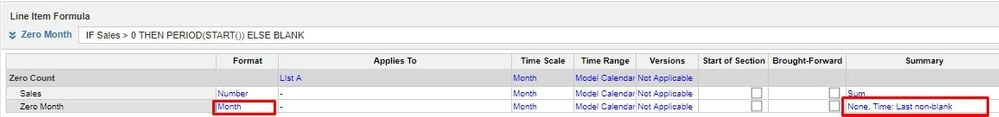Write the below logic in Zero month Line item#2, I have created another module to count the zero month,There are there line item in the module, dimensions are Lists A and Line item

Zero Month = Zero Count.Zero Month[SELECT: TIME.All Periods]

Current Month = PERIOD(CURRENTPERIODSTART())

Count = YEAR(Current Month) * 12 + MONTH(Current Month) - (YEAR(Zero Month) * 12 + MONTH(Zero Month))

~Vignesh

•HI @gregoryTan ,

I have the similar idea as @DavidSmith explained. This will reduce one more line item from the above solution. Please follow the below steps to achieve this.

Step 1: Create a Boolean Flag in your module and make it as Sales <> 0 (Best way to use not equal to to capture the negative values as well).

Step 2 : Create another line item called "Cumulate" and put the formula CUMULATE(1,Boolean Flag)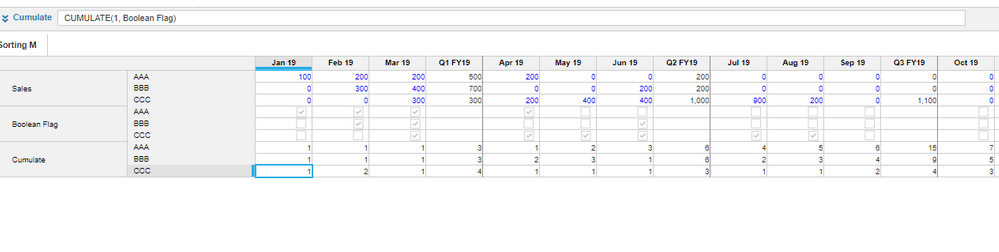Step 3: In your reporting module, Create 2 line items. One it to get the current period and another one is to get the count. Please find the below screen shot for formula refernce.@DavidSmith In this way I think we can optimise the model.

Thanks,

Kavin.

•I always try and split the line items out initially to exaplain the logic, but yes, there is scope for optimisation (Summary options cn be turned off, for example) and I agree with steps 1 and 2

However, in step 3, you should not include the current period line item.  This is inefficient and over calculating,  duplicating the value across the products lists and is not needed.  Use the time lookup module as I outlined

David

•@DavidSmith  I agree 🙂  it is always best practise to use time lookup in seperate module, for the time being I used it in this module.